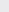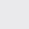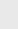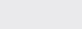Course Content

0/4

0/3

0/1

0/2

0/1

0/4

0/3

0/5

0/3

0/5

0/5

0/1

0/6

0/2

0/4

0/5

11.3 Area of Triangles 三角形的面积

1. Given A(4p, 1), B(2, 5) and C(-2p, 3), find the possible value of p if

(a) the area of triangle ABC is 14 unit,

(b) A, B and C are collinear.

A, B 和 C 共线

(a) 1.5, –2

(b)2. Given that A(-4, -3), B(2, 0) and C(8, -8) are the vertices of a triangle.

(a) Calculate the area of triangle ABC,

(b) Find the length of AC and hence calculate the perpendicular distance from B to AC.

(a) 33unit(b) 13,3. The coordinates of the vertices of a triangle are (3a, a), (2, 3) and (4, 7) in anticlockwise direction. Find the area of the triangle in terms of a. If the area of the triangle is 16 unit, find the value of a.

1 – 5a, –3
4. Given that A, B and C are the three points with coordinates (-3, 8), (2, 3) and (-1, k) respectively where k > 0.
Get the statement of the area of triangle ABC in terms of k. Hence, find the value of k when

(a) the area of triangle ABC is 25 unit,

(b) A, B and C are on the collinear.

A, B 和C 共线。

(a) 16, -4

(b) 6

5. Find the area of the triangle, whose vertices are (2, -1), (t + 1, t – 3), (t + 2, t). Hence show that when t =, these points are collinear.6. Given three vertices of a triangle ABC, A(-2, 2), B(3, 7) and C(4, 0). If the area ofACD andABC are the same andACD = 90, find the coordinate of D.
7. Given that O, A, B are the points (0,0), (1, 2), (4, 0), and C lies on the line x = 2. If the areas ofOAB andOBC are equal, find the coordinates of C.